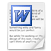### Explanation of the 5x5 (pan)magic square

What is a magic 5x5 square?

The 5x5 magic square is square, because it has as many rows (from left to right = horizontal) as columns (from top to bottom = vertical).

The 5x5 magic square consists of 5 rows which multiplied by 5 columns is 25 cells.

The 5x5 magic square must contain 25 different numbers. A pure magic 5x5 square contains the numbers 1, 2, 3, 4, 5, 6, 7, 8, 9, 10, 11, 12, 13, 14, 15, 16, 17, 18, 19, 20, 21, 22, 23, 24 and 25.

The magic square is magic, because the sum of the numbers of each row, each column and both diagonals always give the same result. The sum can be calculated as follows, the (odd) size of the square multiplied by the middle number: 5 x 13 = 65.

What is a panmagic 5x5 square?

Panmagic (5x5) squares have an extra magic feature. Not only the sum of 5 numbers from each row, each column and both diagonals - but also the sum of 5 numbers from each pandiagonal - totals to the magic sum of 65. Pandiagonals don’t start (unlike ordinary diagonals) in the corner, and pandiagonals are broken diagonals; see below (the ordinary diagonal is yellow and the pandiagonals are red, blue, pink and green):

from right to left                                 from left to right

 1 7 13 19 25 1 7 13 19 25 14 20 21 2 8 14 20 21 2 8 22 3 9 15 16 22 3 9 15 16 10 11 17 23 4 10 11 17 23 4 18 24 5 6 12 18 24 5 6 12

Specialty of the panmagic square is, that you can produce a 2x2 carpet of the same square:

 1 7 13 19 25 1 7 13 19 25 1 7 13 19 25 1 7 13 19 25 14 20 21 2 8 14 20 21 2 8 14 20 21 2 8 14 20 21 2 8 22 3 9 15 16 22 3 9 15 16 22 3 9 15 16 22 3 9 15 16 10 11 17 23 4 10 11 17 23 4 10 11 17 23 4 10 11 17 23 4 18 24 5 6 12 18 24 5 6 12 18 24 5 6 12 18 24 5 6 12 1 7 13 19 25 1 7 13 19 25 1 7 13 19 25 1 7 13 19 25 14 20 21 2 8 14 20 21 2 8 14 20 21 2 8 14 20 21 2 8 22 3 9 15 16 22 3 9 15 16 22 3 9 15 16 22 3 9 15 16 10 11 17 23 4 10 11 17 23 4 10 11 17 23 4 10 11 17 23 4 18 24 5 6 12 18 24 5 6 12 18 24 5 6 12 18 24 5 6 12

Each 5x5 subsquare on the 2x2 carpet is panmagic, because of the parallel running diagonals on the carpet!!!

What is the secret behind the panmagic 5x5 square?

Imagine: A boat sales on a lake of 5 x 5 = 25 square miles. A radar detects the boat and determines in which square mile (= cell) the boat is located. The location of the boat can be expressed in a row coordinate (0, 1, 2, 3, or 4) and a column coordinate (0, 1, 2, 3, or 4).

 All combinations of 0 1 2 3 4 row- and column coordinates 0 (0,0) (1,0) (2,0) (3,0) (4,0) 1 (0,1) ('1,1) (2,1) (3,1) (4,1) 2 (0,2) (1,2) (2,2) (3,2) (4,2) 3 (0,3) (1,3) (2,3) (3,3) (4,3) 4 (0,4) (1,4) (2,4) (3,4) (4,4)

If you add 5x the column coordinate to the row coordinate, you can number the cells from 0 up to 24.

row coordinate                             + 5x column coordinate     =       numbers 0 up to 24

 0 1 2 3 4 10 0 0 0 0 0 0 1 2 3 4 0 1 2 3 4 10 1 1 1 1 1 5 6 7 8 9 0 1 2 3 4 10 2 2 2 2 2 10 11 12 13 14 0 1 2 3 4 10 3 3 3 3 3 15 16 17 18 19 0 1 2 3 4 10 4 4 4 4 4 20 21 22 23 24 10 10 10 10 10

If you use the numbers 0 up to 24 (in stead of 1 up to 25), the magic sum is (the size of the odd square multiplied by the middle number: 5 x 12 = ) 60.

You can also calculate the magic sum as follows: add 5x the sum of the column coordinates (0+1+2+3+4=10) to the sum of the row coordinates (0+1+2+3+4=10): 5x10 + 10 = 60.

If you ensure that all the column coordinates from 0 up to 4 and all the row coordinates from 0 up to 4 are in each row/column/(pan)diagonal, than you will produce everywhere the magic sum of 60 (see calculation mentioned above). Notify that the connection between the column coordinates and the row coordinates must lead to unique column/row-combinations (see table mentioned above), so you can find all the numbers from 0 up to 24 in the magic square.

N.B.: If you add 1 to all numbers, than you get all the numbers from 1 up to 25 in the magic square)

Trick (method)

See for an easy trick using row coordinates and column coordinates to get a panmagic 5x5 square: shift method5x5, explanation.doc
Microsoft Word document 95.0 KB